Next: Class Distributions Up: Continuous Weights Mixture Model Previous: Non-spatial with Class Proportions

### Spatial

We can approximate the distribution in equation 8 by replacing the discrete labels,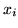, with continuous weights vectors,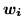: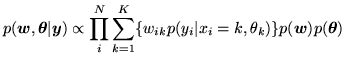(14)

where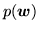, is given by equation 10 with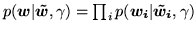where as before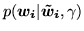is specified by a deterministic mapping betweenand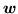(the logistic transform, equation 12).

However, instead of equation 11,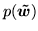is now a continuous Gaussian conditionally specified auto-regressive (CAR) or continuous MRF prior (Cressie, 1993) on each of the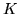class maps, i.e.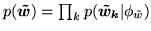(where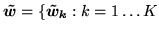} and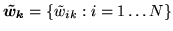) with: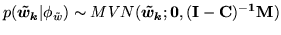(15)

where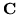is an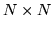matrix whose (i,j)th element is,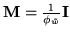, and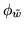is the MRF control parameter which controls the amount of spatial regularisation. We set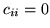,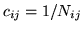if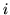and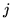are spatial neighbours and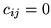otherwise (where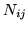is the geometric mean of the number of neighbours for voxelsand) , giving approximately: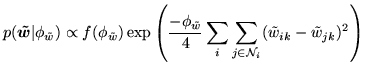(16)

How does this posterior approximation using the continuous class weights vectors instead of class labels allow us to adaptively determine the amount of spatial regularisation? The answer is that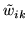is a continuous random variable ranging effectively between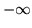and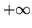. Therefore, unlike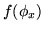in equation 6, the normalising constant,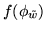, in equation 16 is known: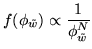(17)

and hence we can adaptively determine the MRF control parameter,. To achieve thisbecomes a parameter in the model, and equation 14 becomes: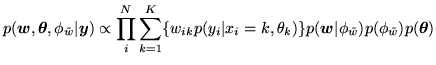(18)

where the prior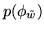, is a non-informative conjugate gamma prior: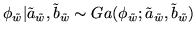(19)

In summary, we have approximated the discrete labels with vectors of continuous weights which a priori approximate delta functions at 0 and 1. Each vector of continuous weights deterministically corresponds (via the logistic transform) to another vector of continuous weights, which a priori are uniform across the real line. As they are uniform across the real line these vectors of continuous weights can be regularised using a spatial prior (a continuous MRF) for which the amount of spatial regularisation can be determined adaptively.

Figure 2 shows a graphical representation of the discrete labels mixture model (equation 8) and the continuous weights spatial mixture model with adaptive spatial regularisation (equation 14).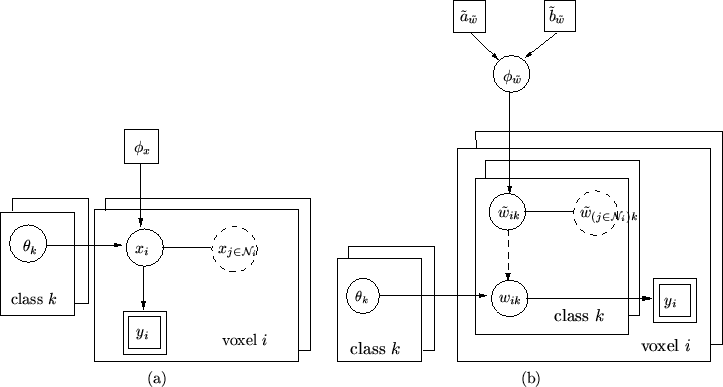Next: Class Distributions Up: Continuous Weights Mixture Model Previous: Non-spatial with Class Proportions